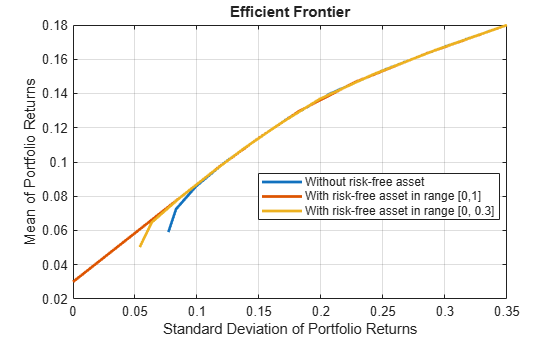Documentation

# setBudget

Set up budget constraints

## Syntax

``obj = setBudget(obj,LowerBudget)``
``obj = setBudget(obj,LowerBudget,UpperBudget)``

## Description

example

````obj = setBudget(obj,LowerBudget)` sets up budget constraints for `Portfolio`, `PortfolioCVaR`, or `PortfolioMAD` objects. For details on the respective workflows when using these different objects, see Portfolio Object Workflow, PortfolioCVaR Object Workflow, and PortfolioMAD Object Workflow.```

example

````obj = setBudget(obj,LowerBudget,UpperBudget)` sets up budget constraints for portfolio objects with an additional option for `UpperBudget`.```

## Examples

collapse all

Assume you have a fund that permits up to 10% leverage, which means that your portfolio can be from 100% to 110% invested in risky assets. Given a Portfolio object `p`, set the budget constraint.

```p = Portfolio; p = setBudget(p, 1, 1.1); disp(p.LowerBudget);```
``` 1 ```
`disp(p.UpperBudget);`
``` 1.1000 ```

Assume you have a fund that permits up to 10% leverage, which means that your portfolio can be from 100% to 110% invested in risky assets. Given a CVaR portfolio object `p`, set the budget constraint.

```p = PortfolioCVaR; p = setBudget(p, 1, 1.1); disp(p.LowerBudget);```
``` 1 ```
`disp(p.UpperBudget);`
``` 1.1000 ```

Assume you have a fund that permits up to 10% leverage, which means that your portfolio can be from 100% to 110% invested in risky assets. Given PortfolioMAD object `p`, set the budget constraint.

```p = PortfolioMAD; p = setBudget(p, 1, 1.1); disp(p.LowerBudget);```
``` 1 ```
`disp(p.UpperBudget);`
``` 1.1000 ```

Define mean and covariance of risk asset returns.

```m = [ 0.05; 0.1; 0.12; 0.18; ]; C = [ 0.0064 0.00408 0.00192 0,; 0.00408 0.0289 0.0204 0.0119,; 0.00192 0.0204 0.0576 0.0336,; 0 0.0119 0.0336 0.1225];```

Create a `Portfolio` object defining the risk-free rate.

```p = Portfolio('RiskFreeRate',0.03, 'assetmean', m, 'assetcovar', C, ... 'lowerbudget', 1, 'upperbudget', 1, 'lowerbound', 0);```

Create multiple `Portfolio` objects with different budgets on risky assets. By defining the risky assets, you can control how much is invested in a risk-free asset.

```p = setBudget(p, 1, 1); % allow 0% risk-free asset allocation, meaning fully invested in risky assets p1 = setBudget(p, 0, 1); % allow 0 to 100% risk-free asset allocation p2 = setBudget(p, 0.7, 1); % allow 0 to 30% risk-free asset allocation plotFrontier(p); hold on; plotFrontier(p1);hold on; plotFrontier(p2); legend('Without risk-free asset', 'With risk-free asset in range [0,1]', 'With risk-free asset in range [0, 0.3]', 'location', 'best');````setBudget` defines the bound for total weights for the allocated risky assets, and the remaining is automatically the bound for a risk-free asset. Use `setBudget` to control the level of allowed allocation to a risk-free asset. For additional information on using `setBudget` with a risk-free asset, see Leverage in Portfolio Optimization with a Risk-Free Asset.

## Input Arguments

collapse all

Object for portfolio, specified using `Portfolio`, `PortfolioCVaR`, or `PortfolioMAD` object. For more information on creating a portfolio object, see

Data Types: `object`

Lower-bound for budget constraint, specified as a scalar for a `Portfolio`, `PortfolioCVaR`, or `PortfolioMAD` input object (`obj`).

### Note

Given bounds for a budget constraint in either `LowerBudget` or `UpperBudget`, budget constraints require any portfolio in `Port` to satisfy:

`LowerBudget <= sum(Port) <= UpperBudget`

One or both constraints may be specified. The usual budget constraint for a fully invested portfolio is to have `LowerBudget = UpperBudget = 1`. However, if the portfolio has allocations in cash, the budget constraints can be used to specify the cash constraints. For example, if the portfolio can hold between 0% and 10% in cash, the budget constraint would be set up with

`obj = setBudget(obj, 0.9, 1)`

Data Types: `double`

Upper-bound for budget constraint, specified as a scalar for a `Portfolio`, `PortfolioCVaR`, or `PortfolioMAD` input object (`obj`).

### Note

Given bounds for a budget constraint in either `LowerBudget` or `UpperBudget`, budget constraints require any portfolio in `Port` to satisfy:

`LowerBudget <= sum(Port) <= UpperBudget`

One or both constraints may be specified. The usual budget constraint for a fully invested portfolio is to have `LowerBudget = UpperBudget = 1`. However, if the portfolio has allocations in cash, the budget constraints can be used to specify the cash constraints. For example, if the portfolio can hold between 0% and 10% in cash, the budget constraint would be set up with

`obj = setBudget(obj, 0.9, 1)`

Data Types: `double`

## Output Arguments

collapse all

Updated portfolio object, returned as a `Portfolio`, `PortfolioCVaR`, or `PortfolioMAD` object. For more information on creating a portfolio object, see

## Tips

You can also use dot notation to set up the budget constraints.

`obj = obj.setBudget(LowerBudget, UpperBudget);`